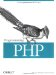3.1 Calling a Function

 Functions in a PHP program can be either built-in (or, by being in an extension, effectively built-in) or user-defined. Regardless of their source, all functions are evaluated in the same way: \$some_value = function_name( [ parameter, ... ] ); The number of parameters a function requires differs from function to function (and, as we'll see later, may even vary for the same function). The parameters supplied to the function may be any valid expression and should be in the specific order expected by the function. A function's documentation will tell you what parameters the function expects and what values you can expect to be returned. Here are some examples of functions: // strlen( ) is a built-in function that returns the length of a string \$length = strlen("PHP"); // \$length is now 3 // sin() and asin( ) are the sine and arcsine math functions \$result = sin(asin(1)); // \$result is the sine of arcsin(1), or 1.0 // unlink( ) deletes a file \$result = unlink("functions.txt"); // false if unsuccessful In the first example, we give an argument, "PHP", to the function strlen( ), which gives us the number of characters in the string it's given. In this case, it returns 3, which is assigned to the variable \$length. This is the simplest and most common way to use a function. The second example passes the result of asin(1) to the sin( ) function. Since the sine and arcsine functions are reflexive, taking the sine of the arcsine of any value will always return that same value. In the final example, we give a filename to the unlink( ) function, which attempts to delete the file. Like many functions, it returns false when it fails. This allows you to use another built-in function, die( ), and the short-circuiting property of the logic operators. Thus, this example might be rewritten as: \$result = unlink("functions.txt") or die("Operation failed!"); The unlink( ) function, unlike the other two examples, affects something outside of the parameters given to it. In this case, it deletes a file from the filesystem. All such side effects of a function should be carefully documented. PHP has a huge array of functions already defined for you to use in your programs. Everything from database access, to creating graphics, to reading and writing XML files, to grabbing files from remote systems can be found in PHP's many extensions. Chapter 14 goes into detail on how to add new extensions to PHP, the built-in functions are described in detail in Appendix A, and an overview of PHP's extensions can be found in Appendix B.Programming PHP
ISBN: 1565926102
EAN: 2147483647
Year: 2007
Pages: 168

Similar book on Amazon